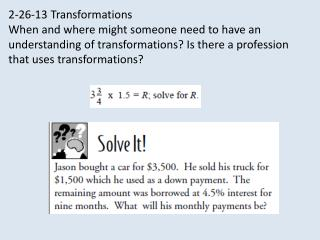DownloadDownload Presentation2-26-13 Transformations

# 2-26-13 Transformations

Télécharger la présentation## 2-26-13 Transformations

- - - - - - - - - - - - - - - - - - - - - - - - - - - E N D - - - - - - - - - - - - - - - - - - - - - - - - - - -
##### Presentation Transcript

1. 2-26-13 Transformations When and where might someone need to have an understanding of transformations? Is there a profession that uses transformations?

2. Check page 745 #1-24 For problems 1-6 You should have given properties to justify each step: Commutative property of addition Additive inverse Associative Property etc. 2(X+1) = 22 OPEN SENTENCE TRUE 45+5 = -50 FALSE 20 60 96 3b+25 3a-6c 5q+2 -17y+38 -3M+39 -x+2 -15 7 -24 23 -67 -22

3. TRANSFORMATIONSin the Coordinate Plane

4. A TRANSFORMATION is when a figure or point is moved to a new position in a coordinate plane. This move may include a change in size as well as position. (Yesterday we worked with dilations which moved points in the plane to enlarge or reduce while creating a similar figure. This was a type of transformation.) A RIGID TRANSFORMATION is when the size and shape remain the same but the figure moves into a new position. Take a moment to add these two descriptions to your vocabulary.

5. There are four types of movement (TRANSFORMATIONS): • DILATION…(Enlarges or Reduces) • TRANSLATION……(Slide) • REFLECTION……..(Flip) • ROTATION…….…..(Turn)

6. Today we will work with TRANSLATIONS Stop and do Translation Activity Once ACTIVITY is complete, we will come back to the powerpoint and add to our notes.

7. D99 Activity

8. http://www.brightstorm.com/math/geometry/transformations/translations/http://www.brightstorm.com/math/geometry/transformations/translations/

9. TRANSLATION is a movement of a figure that involves a slide in the x or y direction on a coordinate plane. More than one move may take place. Here is what a translation may look like. Notice that the direction the triangle is pointing did not change.

10. You have discovered that there are two methods to perform a “TRANSLATION”. Each will give you the new “prime points”.

11. METHOD 1: From each point, conduct the move requested one point at a time and then draw in your new image. Example: Plot points A(-2, 3), B(-6, 3), and C(-2, 7). Translate the figure 8 units right and 5 units down. C STEP 1:Plot original points STEP 2:From each original point move 8 units right and 5 units down. STEP 3:Connect the new points. This is your image and the points are the “prime” points. B A A(-2,3) A’(6, -2) B(-6,3) B’(2, -2) C(-2,7) C’(6, 2) STEP 4: Now list the location of the new points as your “primes”.

12. METHOD 2: A right/left move will affect the x coordinate and an up/down move will affect the y coordinate. You can just do the arithmetic to each part of the ordered pair (coordinates). Example: Plot points A(-2, 3), B(-6, 3), and C(-2, 7). If the figure is translated 8 units right and 5 units down, name the prime points. The 8 units right will add 8 to the x number in the coordinate set and the 5 units down will subtract 5 from the y x y A(-2,3) -2 + 8 = 6 and 3 – 5 = -2 A’(6, -2) B(-6,3) -6 + 8 = 2 and 3 – 5 = -2 B’(2, -2) C(-2,7) -2 + 8 = 6 and 7 – 5 = 2 C’(6, 2)

13. Take a few moments now to add facts to your vocabulary sheet about TRANSLATIONS Handout Translations D100

14. Summary: When and where might someone need to have an understanding of transformations? Is there a profession that uses transformations? Homework: Textbook page 745, #25-39; Handout “Translations Practice”